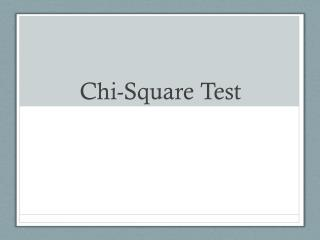DownloadDownload PresentationChi-Square Test

# Chi-Square Test

Télécharger la présentation## Chi-Square Test

- - - - - - - - - - - - - - - - - - - - - - - - - - - E N D - - - - - - - - - - - - - - - - - - - - - - - - - - -
##### Presentation Transcript

1. Chi-Square Test

2. Chi-Square (χ2) Test • Used to determine if there is a significant difference between the expected and observed data • Null hypothesis: There is NO statistically significant difference between expected & observed data • Any differences are due to CHANCE alone

3. Chi-Square (χ2) Formula

4. How to use the Chi-Square Test • Determine null hypothesis • All frequencies are equal –OR– Specific frequencies given already • Use formula to calculate χ2 value: • n = # of categories,e = expected, o = observed • Find critical value using table (Use p=0.05). • degrees of freedom (df) = n – 1 • If χ2 < Critical Value, then ACCEPT null hypothesis. Differences in data are due to chance alone. If χ2 > Critical Value, REJECT the null hypothesis: Differences in data are NOT due to chance alone!

5. Sample Problem • You buy a package of M&Ms from the factory store and find the following: 20 brown, 20 blue, 20 orange, 20 green, and 20 yellowM&Ms. • According to the M&M website, each package of candy should have 13% brown, 24% blue, 20% orange, 16% green, 13% red, and 14% yellowM&Ms. • You realize you are missing Red M&M’s in your package! Is this acceptable, or did something happen in the factory during the packaging process? • Use the Chi-Square Test to answer this question.